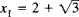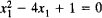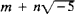# Algebraic Integer

(redirected from Algebraic integers)

## algebraic integer

[¦al·jə¦brā·ik ′in·tə·jər]
(mathematics)
The root of a polynomial whose coefficients are integers and whose leading coefficient is equal to 1.
McGraw-Hill Dictionary of Scientific & Technical Terms, 6E, Copyright © 2003 by The McGraw-Hill Companies, Inc.
The following article is from The Great Soviet Encyclopedia (1979). It might be outdated or ideologically biased.

## Algebraic Integer

a number that is a root of an equation of the form xn + a1xn – 1 + · · · + an = 0, where a1,..., an are integers. For example,is an algebraic integer, since.

The theory of algebraic integers developed in the 1830’s and 1840’s as a result of the work of K. Jacobi, F. Eisenstein, and E. Kummer on reciprocity laws of higher degree, on Fermat’s theorem, and on the generalization of the arithmetic of complex integers.

The sum, difference, and product of algebraic integers are algebraic integers; that is, the set of algebraic integers forms a ring. The theory of the divisibility of algebraic integers, however, differs from the theory of the divisibility of ordinary integers. Algebraic integers of the form, where m and n are ordinary integers, are discussed in IDEAL.

References in periodicals archive ?
Dubickas, Additive Hilbert's Theorem 90 in the ring of algebraic integers, Indag.
Rumely's introduction to this exposition on the Fekete-Szego theorem includes a sketch of the basic proof and contextualizes its development with the Robinson theorem on totally real algebraic integers in an interval and Cantor's extension with splitting conditions.
Encoding real numbers to AI representation involves a mapping function [f.sub.enc](x; z) = a, where x is a real number, z is a fixed array of algebraic integers, and a is an array of integers, which represents x in the AI basis.
Miller, "New DCT algorithm based on encoding algebraic integers," in Proceedings of the IEEE International Conference on Acoustics, Speech and Signal Processing (ICASSP '98), vol.
Algebraic integer (AI) encoding  has been proposed for addressing this problem.
Dimitrov, "Systolic implementation of real-valued discrete transforms via algebraic integer quantization," Computers and Mathematics with Applications, vol.
On the other side, algebraic integers lying in an extension field of the rationals are generated with the same linear polynomials.
Therefore Z[[[xi].sub.n] + [xi].sup.-1.sub.n]] is the ring of algebraic integers in the field Q([[[xi].sub.n] + [xi].sup.-1.sub.n]]).
Let [theta] be an algebraic integer, and f (x) the minimal polynomial of 9 over Q.
Their topics include divisibility, polynomial congruences, quadratic reciprocity, the geometry of numbers, and algebraic integers. An epilogue (of course) considers Fermat's last theorem.
The field F will be called norm-Euclidean if, for every pair ([alpha],[beta]) of algebraic integers in F with [beta] [not equal to] 0, there exists an algebraic integer [gamma] in F such that [absolute value of ([N.sub.F]([alpha] - [gamma][beta]))] < [absolute value of ([N.sub.F]([beta]))].
Let 1 be the prime ideal of F' dividing l, and [lambda] an algebraic integer in F' generating the principal ideal 1.

Site: Follow: Share:
Open / Close